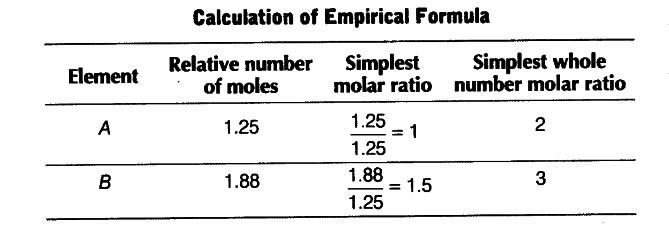# A compound made up of two elements A and B

A compound made up of two elements A and B has A = 70%, B = 30%. Their relative number of moles in the compound are 1.25 and 1.88. Calculate
(i) atomic masses of the elements A and B
(ii) molecular formula of the compound, if its molecular mass is found to be 160.

Relative number of moles of an element = % of the element / atomic mass
or atomic mass = % of element / relative number of moles
Atomic mass of A = 70 / 1,25 = 56

and Atomic mass of B = 30 / 1.88 =16Empirical formula = \$A _{ 2 }\$\$B _{ 3 } \$
Calculation of molecular formula

Empirical formula mass = 2 x 56 + 3 x 16 = 160

n = molecular mass / empirical formula mass = 160/160 = 1
Molecular formula = \$A _{ 2 }\$\$B _{ 3 } \$